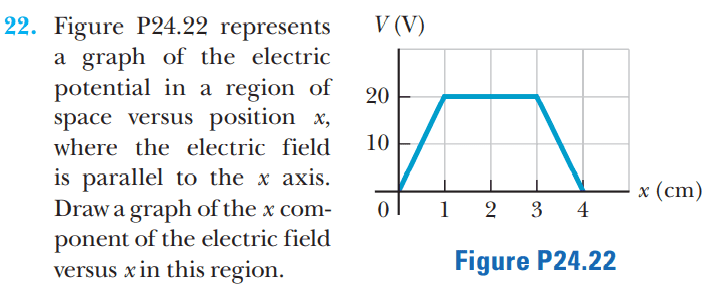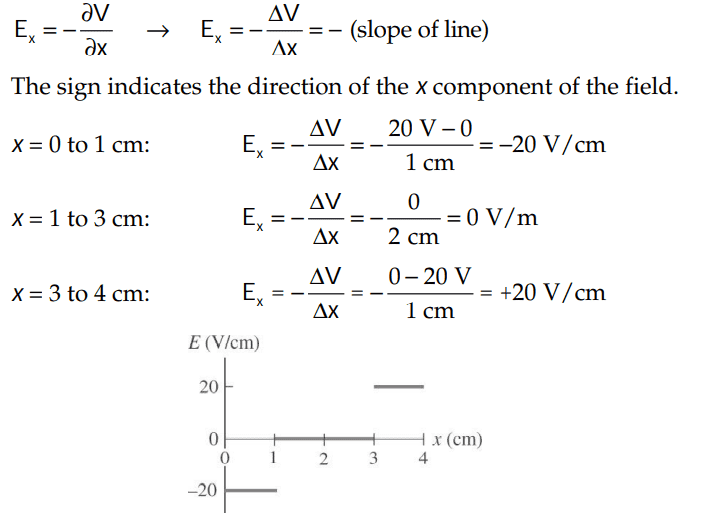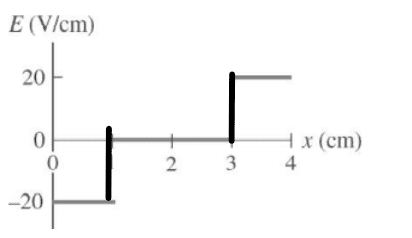# Drawing the Graph: Analyzing Solutions and Questions

• ChiralSuperfields

#### ChiralSuperfields

Homework Statement
Relevant Equations
For this problem,,
The solutions is,However, why did they not draw vertical lines for the graph like this:?

Thank you!

However, why did they not draw vertical lines for the graph like this:
Because the electric field is discontinuous at x = 1 cm and x = 3 cm and that is how discontinuous functions are plotted. Drawing a line as you suggest implies that the electric field has all the in-between values which it doesn't.

•ChiralSuperfields
Because the electric field is discontinuous at x = 1 cm and x = 3 cm and that is how discontinuous functions are plotted. Drawing a line as you suggest implies that the electric field has all the in-between values which it doesn't.
Got it, thank you @kuruman !